Main Content

# Loma Prieta Earthquake Analysis

This example shows how to analyze and visualize earthquake data.

### Load Earthquake Data

The file `quake.mat` contains 200Hz data from the October 17, 1989 Loma Prieta earthquake in the Santa Cruz Mountains. The data are courtesy of Joel Yellin at the Charles F. Richter Seismological Laboratory, University of California, Santa Cruz.

Start by loading the data.

```load quake whos e n v```
``` Name Size Bytes Class Attributes e 10001x1 80008 double n 10001x1 80008 double v 10001x1 80008 double ```

In the workspace there are three variables, containing time traces from an accelerometer located in the Natural Sciences building at UC Santa Cruz. The accelerometer recorded the main shock amplitude of the earthquake wave. The variables `n`, `e`, `v` refer to the three directional components measured by the instrument, which was aligned parallel to the fault, with its N direction pointing in the direction of Sacramento. The data are uncorrected for the response of the instrument.

Create a variable, `Time`, containing the timestamps sampled at 200Hz with the same length as the other vectors. Represent the correct units with the `seconds` function and multiplication to achieve the Hz (${\mathit{s}}^{-1}$) sampling rate. This results in a `duration` variable which is useful for representing elapsed time.

```Time = (1/200)*seconds(1:length(e))'; whos Time```
``` Name Size Bytes Class Attributes Time 10001x1 80010 duration ```

### Organize Data in Timetable

Separate variables can be organized in a `table` or `timetable` for more convenience. A `timetable` provides flexibility and functionality for working with time-stamped data. Create a `timetable` with the time and three acceleration variables and supply more meaningful variable names. Display the first eight rows using the `head` function.

```varNames = {'EastWest', 'NorthSouth', 'Vertical'}; quakeData = timetable(Time, e, n, v, 'VariableNames', varNames); head(quakeData)```
```ans=8×3 timetable Time EastWest NorthSouth Vertical _________ ________ __________ ________ 0.005 sec 5 3 0 0.01 sec 5 3 0 0.015 sec 5 2 0 0.02 sec 5 2 0 0.025 sec 5 2 0 0.03 sec 5 2 0 0.035 sec 5 1 0 0.04 sec 5 1 0 ```

Explore the data by accessing the variables in the `timetable` with dot subscripting. (For more information on dot subscripting, see Access Data in a Table.) We chose "East-West" amplitude and `plot` it as function of the duration.

```plot(quakeData.Time,quakeData.EastWest) title('East-West Acceleration')```### Scale Data

Scale the data by the gravitational acceleration, or multiply each variable in the table by the constant. Since the variables are all of the same type (`double`), you can access all variables using the dimension name, `Variables`. Note that `quakeData.Variables` provides a direct way to modify the numerical values within the timetable.

`quakeData.Variables = 0.098*quakeData.Variables;`

### Select Subset of Data for Exploration

We are interested in the time region where the amplitude of the shockwave starts to increase from near zero to maximum levels. Visual inspection of the above plot shows that the time interval from 8 to 15 seconds is of interest. For better visualization we draw black lines at the selected time spots to draw attention to that interval. All subsequent calculations will involve this interval.

```t1 = seconds(8)*[1;1]; t2 = seconds(15)*[1;1]; hold on plot([t1 t2],ylim,'k','LineWidth',2) hold off```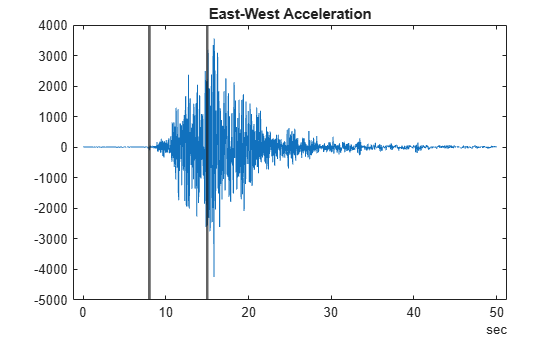### Store Data of Interest

Create another `timetable` with data in this interval. Use `timerange` to select the rows of interest.

```tr = timerange(seconds(8),seconds(15)); dataOfInterest = quakeData(tr,:); head(dataOfInterest)```
```ans=8×3 timetable Time EastWest NorthSouth Vertical _________ ________ __________ ________ 8 sec -0.098 2.254 5.88 8.005 sec 0 2.254 3.332 8.01 sec -2.058 2.352 -0.392 8.015 sec -4.018 2.352 -4.116 8.02 sec -6.076 2.45 -7.742 8.025 sec -8.036 2.548 -11.466 8.03 sec -10.094 2.548 -9.8 8.035 sec -8.232 2.646 -8.134 ```

Visualize the three acceleration variables on three separate axes.

```figure subplot(3,1,1) plot(dataOfInterest.Time,dataOfInterest.EastWest) ylabel('East-West') title('Acceleration') subplot(3,1,2) plot(dataOfInterest.Time,dataOfInterest.NorthSouth) ylabel('North-South') subplot(3,1,3) plot(dataOfInterest.Time,dataOfInterest.Vertical) ylabel('Vertical')```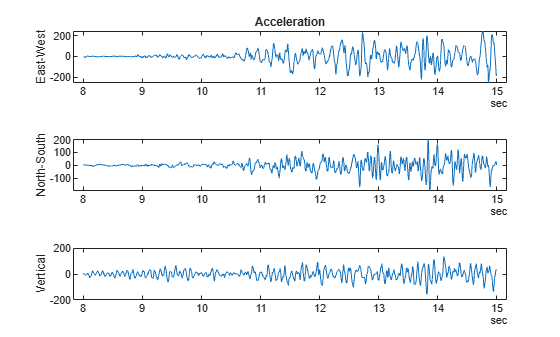### Calculate Summary Statistics

To display statistical information about the data we use the `summary` function.

`summary(dataOfInterest)`
```RowTimes: Time: 1400x1 duration Values: Min 8 sec Median 11.498 sec Max 14.995 sec TimeStep 0.005 sec Variables: EastWest: 1400x1 double Values: Min -255.09 Median -0.098 Max 244.51 NorthSouth: 1400x1 double Values: Min -198.55 Median 1.078 Max 204.33 Vertical: 1400x1 double Values: Min -157.88 Median 0.98 Max 134.46 ```

Additional statistical information about the data can be calculated using `varfun`.This is useful for applying functions to each variable in a table or timetable. The function to apply is passed to `varfun` as a function handle. Below we apply the `mean` function to all three variables and output the result in format of a table, since the time is not meaningful after computing the temporal means.

`mn = varfun(@mean,dataOfInterest,'OutputFormat','table')`
```mn=1×3 table mean_EastWest mean_NorthSouth mean_Vertical _____________ _______________ _____________ 0.9338 -0.10276 -0.52542 ```

### Calculate Velocity and Position

To identify the speed of propagation of the shockwave, we integrate the accelerations once. We use cumulative sums along the time variable to get the velocity of the wave front.

```edot = (1/200)*cumsum(dataOfInterest.EastWest); edot = edot - mean(edot);```

Below we perform the integration on all three variables to calculate the velocity. It is convenient to create a function and apply it to the variables in the `timetable` with `varfun`. In this example, we included the function at the end of this file and named it `velFun`.

```vel = varfun(@velFun,dataOfInterest); head(vel)```
```ans=8×3 timetable Time velFun_EastWest velFun_NorthSouth velFun_Vertical _________ _______________ _________________ _______________ 8 sec -0.56831 0.44642 1.8173 8.005 sec -0.56831 0.45769 1.834 8.01 sec -0.5786 0.46945 1.832 8.015 sec -0.59869 0.48121 1.8114 8.02 sec -0.62907 0.49346 1.7727 8.025 sec -0.66925 0.5062 1.7154 8.03 sec -0.71972 0.51894 1.6664 8.035 sec -0.76088 0.53217 1.6257 ```

Apply the same function `velFun` to the velocities to determine the position.

```pos = varfun(@velFun,vel); head(pos)```
```ans=8×3 timetable Time velFun_velFun_EastWest velFun_velFun_NorthSouth velFun_velFun_Vertical _________ ______________________ ________________________ ______________________ 8 sec 2.1189 -2.1793 -3.0821 8.005 sec 2.1161 -2.177 -3.0729 8.01 sec 2.1132 -2.1746 -3.0638 8.015 sec 2.1102 -2.1722 -3.0547 8.02 sec 2.107 -2.1698 -3.0458 8.025 sec 2.1037 -2.1672 -3.0373 8.03 sec 2.1001 -2.1646 -3.0289 8.035 sec 2.0963 -2.162 -3.0208 ```

Notice how the variable names in the timetable created by `varfun` include the name of the function used. It is useful to track the operations that have been performed on the original data. Adjust the variable names back to their original values using dot notation.

`pos.Properties.VariableNames = varNames;`

Below we plot the 3 components of the velocity and position for the time interval of interest.

```figure subplot(2,1,1) plot(vel.Time,vel.Variables) legend(quakeData.Properties.VariableNames,'Location','NorthWest') title('Velocity') subplot(2,1,2) plot(vel.Time,pos.Variables) legend(quakeData.Properties.VariableNames,'Location','NorthWest') title('Position')```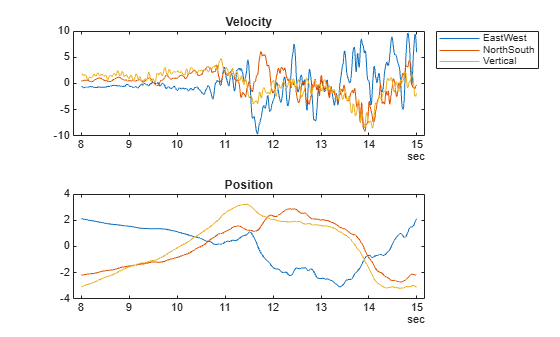### Visualize Trajectories

The trajectories can be plotted in 2D or 3D by using the component value. In the following we will show different ways of visualizing this data.

Begin with 2-dimensional projections. Here is the first with a few values of time annotated.

```figure plot(pos.NorthSouth,pos.Vertical) xlabel('North-South') ylabel('Vertical') % Select locations and label nt = ceil((max(pos.Time) - min(pos.Time))/6); idx = find(fix(pos.Time/nt) == (pos.Time/nt))'; text(pos.NorthSouth(idx),pos.Vertical(idx),char(pos.Time(idx)))```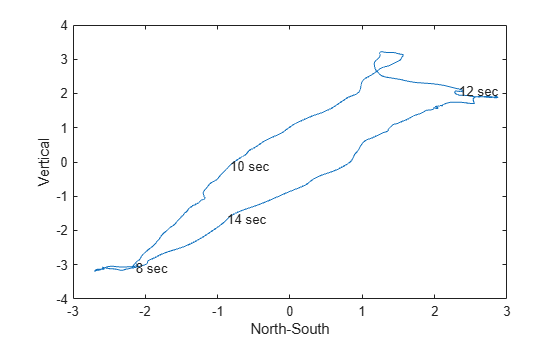Use `plotmatrix` to visualize a grid of scatter plots of all variables against one another and histograms of each variable on the diagonal. The output variable `Ax`, represents each axes in the grid and can be used to identify which axes to label using `xlabel` and `ylabel`.

```figure [S,Ax] = plotmatrix(pos.Variables); for ii = 1:length(varNames) xlabel(Ax(end,ii),varNames{ii}) ylabel(Ax(ii,1),varNames{ii}) end```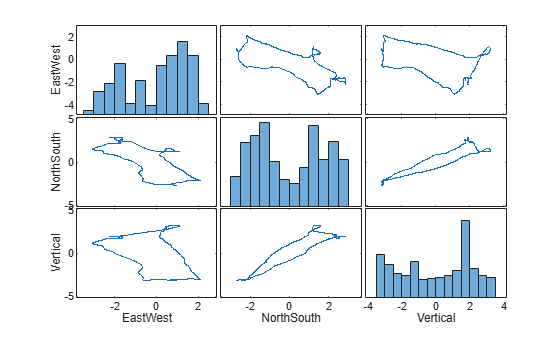Plot a 3-D view of the trajectory and plot a dot at every tenth position point. The spacing between dots indicates the velocity.

```step = 10; figure plot3(pos.NorthSouth,pos.EastWest,pos.Vertical,'r') hold on plot3(pos.NorthSouth(1:step:end),pos.EastWest(1:step:end),pos.Vertical(1:step:end),'.') hold off box on axis tight xlabel('North-South') ylabel('East-West') zlabel('Vertical') title('Position')```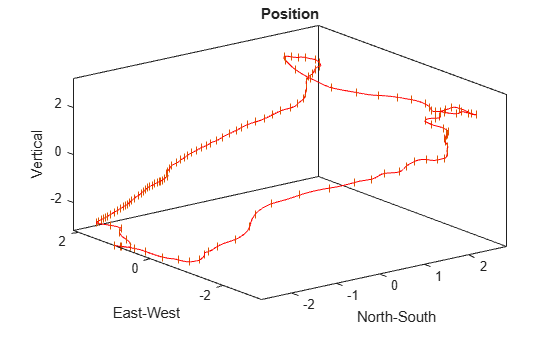### Supporting Functions

Functions are defined below.

```function y = velFun(x) y = (1/200)*cumsum(x); y = y - mean(y); end```

Download ebook